# Texas Go Math Grade 4 Lesson 7.5 Answer Key Multiply Using Partial Products

Refer to our Texas Go Math Grade 4 Answer Key Pdf to score good marks in the exams. Test yourself by practicing the problems from Texas Go Math Grade 4 Lesson 7.5 Answer Key Multiply Using Partial Products.

## Texas Go Math Grade 4 Lesson 7.5 Answer Key Multiply Using Partial Product

Essential Question

How can you use place value and partial products to multiply by a 1-digit number?

Unlock the problem

Connect How can you use what you know about the Distributive Property to break apart numbers to find products of 3-digit and 1-digit numbers?

• How can you write 182 as a sum of hundreds, tens, and ones?
One hundred Eight tens and Two ones.

Explanation:
We can write 182 as a sum of hundreds, tens, and ones as one hundred eight tens and two ones.

Use place value and partial products.
Multiply. 6 × 182 Estimate. 6 × 200 = ________________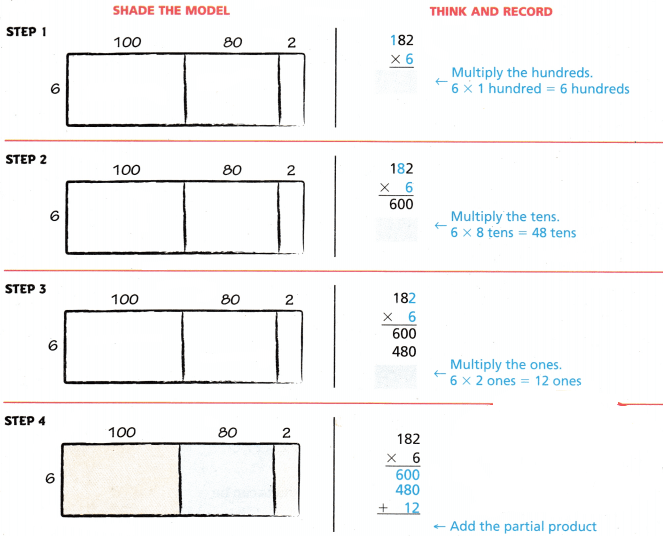Math Talk

Mathematical processes
How can you use the Distributive Property to find 4 × 257?
4 × 257 = 1,028.

Explanation:
Here, Distributive property means if we multiply a sum by a number is the same as multiplying each addend by the number and adding the products. So by distributive property 4 × 257 is
4 × 257 = 4 × (200+50+7)
= (4 × 200) + (4 × 50) + (4 × 7)
= 800+200+28
= 1,028.

Share and Show

Question 1.
Use the model to find 2 × 137.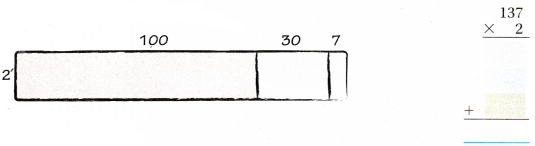2 × 137 = 274.

Explanation:
Here, Distributive property means if we multiply a sum by a number is the same as multiplying each addend by the number and adding the products. So by distributive property 2 × 137 is
2 × 137 = 2 × (100+30+7)
= (2 × 100) + (2 × 30) + (2 × 7)
= 200+60+14
= 274.

Estimate. Then record the product.

Question 2.
Estimate: ___________The product of 190×3 = 570,
Estimated is 200×3 is 600.

Explanation:
Here, we will round off 190 to 200, so 200×3 is 600. And the product of 190×3 is 570.

Question 3.
Estimate: ___________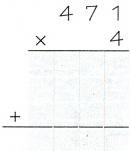The product of 471×4 = 1,884,
Estimated is 500×4 is 2000.

Explanation:
Here, we will round off 471 to 500, so 500×4 is 2000. And the product of 471×4 is 1,884.

Question 4.
Estimate: ___________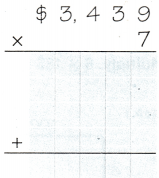The product of 3,439×7 = 24,073,
Estimated is 3,500×7 is 24,500.

Explanation:
Here, we will round off 3,439 to 3,500, so 3,500×7 is 24,500. And the product of 3,439×7 is 24,073.

Math Talk

Mathematical Processes
Explain how using place value and expanded form makes it easier to find products.
By expanded form we can understand better and rightly read the higher digit numbers. And by the place value, we can make sure that the digits are in the correct place.

Problem Solving

Practice: Copy and Solve Estimate. Then record the product.

Question 5.
2 × 78
The product of 78×2 = 156,
Estimated is 100×2 is 200.

Explanation:
Here, we will round off 78 to 100, so 100×2 is 200. And the product of 78×2 is 156.

Question 6.
2 × $210 Answer: The product of$210×2 = $420, Estimated is$200×2 is $400. Explanation: Here, we will round off 210 to 200, so 200×2 is 400. And the product of 210×2 is 420. Question 7. 9 ×$682
The product of $682×9 = 6,138, Estimated is$700×9 is 6,300.

Explanation:
Here, we will round off $682 to$700, so $700×9 is 6,300. And the product of$682×9 is 6,138.

Question 8.
8 × 8,145
The product of 8,145×8 = 65,160,
Estimated is 8,000×8 is 64,000.

Explanation:
Here, we will round off 8,145 to 8,000, so 8,000×8 is 64,000. And the product of 8,145×8 is 65,160.

H.O.T. Algebra Find the missing digit.

Question 9.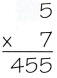The missing digit is 6.

Explanation:
Given the product is 455 and the multiplicand is 7, here we will divide the product with multiplicand so that we can get the missing digit. So 455÷7 which is 65, so the missing digit is 6.

Question 10.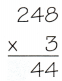The missing digit is 7.

Explanation:
Here, we will perform multiplication to know the missing value, so 248×3 = 744. So the missing digit is 7.

Question 11.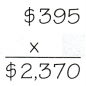The missing digit is 6.

Explanation:
Given the product is $2,370 and the multiplier is$395, here we will divide the product with multiplier so that we can get the missing digit. So $2,370÷$395 which is 6, so the missing digit is 6.

Question 12.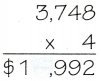The missing digit is 4.

Explanation:
Here, we will perform multiplication to know the missing value, so 3,748×4 = 14,992. So the missing digit is 4.

Question 13.
Write Math Explain how modeling partial products can be used to find the products of greater numbers.
Here, modeling partial products can be used to find the products of greater numbers as the multiplication is easier. So for example if we multiiply
48×3 = (40+8)×3
= 40×3 + 8×3
= 120+24
= 144.

Problem Solving

Question 14.
Look at the picture. Kylie has 832 songs ‘ on her portable media player. Lance has 3 times as many songs. How many fewer songs can Lance add to his player than Kylie can add to hers?Lance can add 1664 fewer songs to his player than Kylie.

Explanation:
Given that the total number of songs in portable media players are 9,000, and kylie has 832 songs. So Kylie can add 9000-832= 8,168 songs. Lance has 3 times as many songs as Kylie, So Lance has 832×3= 2,496. He can add 9000-2496= 6504 to his player. Therefore 8168-6504=1664. Lance can add 1664 fewer songs to his player than Kylie.

Question 15.
Multi-Step James wants to buy the new portable media player shown. He has 5 times as many songs as Susan. Susan has 1,146 songs. Will all of his songs fit on the portable media player? How many songs does James have?
James has 5,730 songs and those all songs can fit on the portable media player.

Explanation:
Given that Susan has 1,146 songs and James has 5 times as many songs as Susan, so 1,146×5= 5,730 songs will fit on the portable media player.

Question 16.Multi-Step
The sum of a 3-digit number and a 1-digit number is 217. The product of the numbers is 642. If one number is between 200 and 225, what are the numbers?
The number is 3.

Explanation:
Given that the product is 642 and the 3 digit number is between 200 and 225, So the 1 digit number is 3 because if we multiply 200 and 225 by 3 we will get the product as 600 and 675 and 642 is in between them. So 642 3= 214. And the one-digit number is 3.

Question 17.
H.O.T. What’s the Error? Hal says that the greatest product of a 3-digit number and a 1-digit number is 8,891. Is he correct? Explain.
Here, Hal says that the greatest product of a 3-digit number and a 1-digit number is 8,891, he is not correct as 999 times 9 is 8,991 but Hal says that 8,891, so he is wrong.

Question 18.
A guide charges $128 to take a group of people on a tour of an island. How much will the guide charge to take 4 groups of the same size on tours? (A)$256
(B) $412 (C)$192
(D) $512 Answer: D. Explanation: Given that a guide charges$128 to take a group of people on a tour of an island, so the guide will charge to take 4 groups of the same size on tours is $128×4 which is$512.

Question 19.
Kalista works 164 hours each month. How many hours does she work in 8 months?
(A) 1,282 hours
(B) 1,312 hours
(C) 1,476 hours
(D) 1,600 hours
B.

Explanation:
Given that Kalista works 164 hours each month. So for 8 months it will be 164×8 which is 1,312 hours.

Question 20.
Multi-Step A trip from El Paso to Dallas, Texas, is 638 miles. A trip from Dallas to Atlanta, Georgia, is 780 miles. If you always go through Dallas, how long is a trip from El Paso to Atlanta and then back to El Paso?
(A) 1,418miles
(B) 1,560 miles
(C) 2,836miles
(D) 3,226 miles
C.

Explanation:
Given that a trip from El Paso to Dallas, Texas, is 638 miles and a trip from Dallas to Atlanta, Georgia, is 780 miles. So the trip from El Paso to Atlanta and then back to El Paso is
2×(638+780) = 2×(1,418)
= 2,836 miles.

TEXAS Test Prep

Question 21.
Mrs. Jackson bought 6 gallons of juice for a party. Each gallon has 16 cups. If 3 cups of juice were left over, how many cups did people drink at the party?
(A) 93 cups
(B) 48 cups
(C) 78 cups
(D) 13 cups
A.

Explanation:
Given that Mrs. Jackson bought 6 gallons of juice for a party and each gallon has 16 cups which is 6×16 = 96 cups. And if 3 cups of juice were left over, so the number of cups did people drink at the party is 96-3 = 93 cups.

### Texas Go Math Grade 4 Lesson 7.5 Homework and Practice Answer Key

Estimate. Then record the product.

Question 1.
Estimate: _____________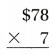The product of $78×7 =$546,
Estimated is $100×7 is$700.

Explanation:
Here, we will round off 78 to 100, so $100×7 is$700. And the product of $78×7 is$546.

Question 2.
Estimate: _____________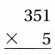The product of 351×5 = 1,755,
Estimated is 400×5 is 2000.

Explanation:
Here, we will round off 351 to 400, so 400×5 is 2000. And the product of 351×5 is 1,755.

Question 3.
Estimate: _____________The product of 2,209×4 = 8,836,
Estimated is 2,200×4 is 8,800.

Explanation:
Here, we will round off 2,209 to 2,200, so 2,200×4 is 8,800. And the product of 2,209×4 is 8,836.

Question 4.
3 × 56
The product of 56×3 = 168,
Estimated is 100×3 is 300.

Explanation:
Here, we will round off 56 to 100, so 100×3 is 300. And the product of 56×3 is 168.

Question 5.
6 × $315 Answer: The product of$315×6 = 1,890,
Estimated is $300×6 is 1,800. Explanation: Here, we will round off$315 to $300, so$300×6 is $1,800. And the product of$315×6 is 1,890.

Question 6.
8 × 1,253
The product of 1,253×8 = 10,024,
Estimated is 1,300×8 is 10,400.

Explanation:
Here, we will round off 1,253 to 1,300, so 1,300×8 is 10,400. And the product of 1,253×8 is 10,024.

Find the missing digit.

Question 7.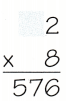The missing digit is 7.

Explanation:
Given the product is 576 and the multiplicand is 7, here we will divide the product with multiplicand so that we can get the missing digit. So 576÷8 which is 72, so the missing digit is 7.

Question 8.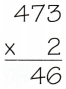The missing digit is 9.

Explanation:
Here, we will perform multiplication to know the missing value, so 473×2 = 946. So the missing digit is 9.

Question 9.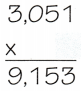The missing digit is 3.

Explanation:
Given the product is 9,153 and the multiplier is 3,051, here we will divide the product with multiplier so that we can get the missing digit. So 9,153÷3,051 which is 3, so the missing digit is 3.

Problem Solving

Four friends are having a contest. They will see who is the first to load 8,000 Songs on their media player.

Question 10.
Jeannie has loaded 467 songs. Paul has loaded 4 times as many songs as Jeannie. how many more songs has Paul loaded than Jeannie?
The number of songs has Paul loaded than Jeannie is 1,868 songs.

Explanation:
Given that Jeannie has loaded 467 songs and Paul has loaded 4 times as many songs as Jeannie, so the number of songs has Paul loaded than Jeannie is 467×4 which is 1,868 songs.

Question 11.
Juro has loaded 1,632 songs. Jessica has loaded 5 times as many. How many songs does Jessica have? Is it enough to win the contest? Explain.
The number of songs does Jessica have is 8,160 songs.

Explanation:
Given that Juro has loaded 1,632 songs and Jessica has loaded 5 times as many. So the number of songs does Jessica have is 1,632×5 which is 8,160 songs. Yes he won the contest.

Lesson Check

Question 12.
A store bought 9 cases of light bulbs. There 13. are 48 light bulbs in a case. How many light bulbs does the store buy?
(A) 108
(B) 432
(C) 362
(D) 392
B.

Explanation:
Given that a store bought 9 cases of light bulbs and there 13, 48 light bulbs in a case. So the number of light bulbs does the store buy 48×9 which is 432.

Question 13.
The Wilson family is flying to Dallas for a wedding. Airplane tickets cost $372 each. How much will 6 tickets cost? (A)$1,854
(B) $2,022 (C)$1,612
(D) $2,232 Answer: D. Explanation: Given that the Wilson family is flying to Dallas for a wedding and airplane tickets cost$372 each. So the cost of 6 tickets cost is $372×6 which is$2,232.

Question 14.
Hugo drives 208 miles to and from work each week. How many miles does he drive in 4 weeks?
(A) 112 miles
(B) 1,120 miles
(C) 832 miles
(D) 8,032 miles
C.

Explanation:
Given that Hugo drives 208 miles to and from work each week. So the number of miles does he drive in 4 weeks is 208×4 which is 832 miles.

Question 15.
Which is the best estimate of the product of 4,306 × 7?
(A) 2,800
(B) 28,000
(C) 2,100
(D) 35,000
D.

Explanation:
Given that the best estimate of the product of 4,306×7 is 35,000. As we will round off 4,306 to 4,500.

Question 16.
Multi-Step Coach Ramirez bought 8 cases of bottled water for a road race. There are 24 bottles in each case. After the race, 34 bottles of water were left. How many bottles were used at the race?
(A) 192
(B) 158
(C) 248
(D) 10
B.

Explanation:
Given that Coach Ramirez bought 8 cases of bottled water for a road race and there are 24 bottles in each case which is 24×8=192 bottles. After the race, 34 bottles of water were left, so the number of bottles were used at the race is 192-34 = 158 bottles.

Question 17.
Multi-Step The sum of a three-digit number and a one-digit number is 160. The product of the two numbers is 624. What are the two numbers?
(A) 156 and 4
(B) 151 and 9
(C) 78 and 8
(D) 155 and 5